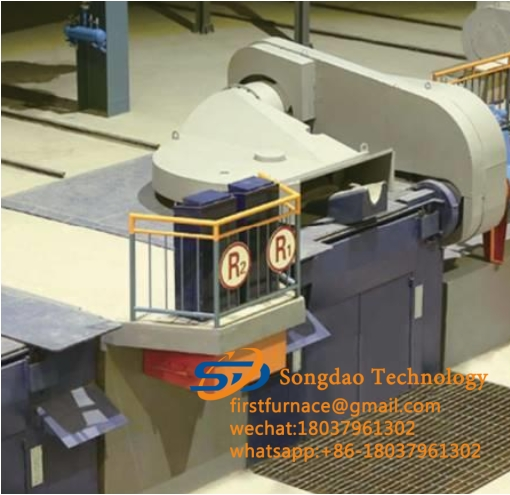### Induction melting furnace power calculation

Induction melting furnace power calculation

1. Parameter calculation of thyristor

The power of the steel pipe heating furnace is 1500KW, and the designed incoming line voltage is 500V. After calculation, the following data can be obtained.

DC voltage Ud=1.35×500=675V

DC current Id=1500000÷675=2200A

Intermediate frequency voltage US=1.5×Ud =1000V

Rated silicon rectifier current IF=0.38×Id÷2÷0.85=491A

(The division by 2 in the above formula is because there are two sets of identical rectifier parts)

Rated silicon rectifier voltage UV=1.414×UL=1.414×500=707V

Inverter silicon rated current IF=Id/2=1100A

Inverter silicon rated voltage UV=1.414×US=1414V

2. Selection scheme of SCR model

The rectifier SCR selects KP1500A/2000V, that is, the rated current is 1500A, and the rated voltage is 2000V. Compared with the theoretical value, the voltage margin is 2.26 times, and the current margin is 2.43 times.

The inverter thyristor KK2500A/2000V of the intermediate frequency power supply, that is, the rated current is 2500A, and the rated voltage is 2000V. In addition, the inverter silicon is connected to the inverter bridge in a double-silicon series connection, so that the actual rated voltage of the thyristor on each inverter bridge arm is 5000V. Compared with the theoretical value, the voltage margin is 2.26 times, and the current margin is 2.15 times.

3. IF resonant capacitor cabinet

The intermediate frequency resonant capacitors of this set of capacitor cabinets are all electrothermal capacitors produced by Xin’anjiang Power Capacitor Factory, the model is RFM2 1.0 -2000-1.0S. Its capacity is 2000KVar, and the operating frequency is 1000Hz.

4. The power of the induction melting furnace is calculated as pw=DC voltage×DC current